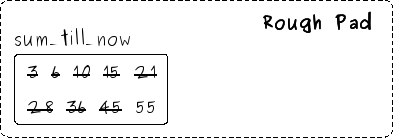Wednesday, October 22, 2008

Learning Computer Programming - Page 2

Let's write a program for adding the numbers from 1 to 10 (i. e. 1 + 2 + 3 + 4 + 5 + 6 + 7 + 8 + 9 + 10).

Let's first try to do it ourselves, manually. Be patient not to miss a single step written below. Every word below is quite important.

We shall be using a rough pad and pencil. The rough pad will be used to scribble the intermediate results that we need to remember temporarily while solving the problem. As you shall see, in stead of scribbling on the pad directly, we shall be drawing some boxes and write inside the boxes. We shall give unique names to the boxes so that we can refer those.

So, start following the steps below.

Add 1 and 2 and note the sum in a box in your rough pad. Name the box as, say, sum_till_now. Or you can choose any name you like. So, your rough pad now looks like this:Add 3 to the value stored in sum_till_now, and write the result back in sum_till_now. Note that as the box sum_till_now can contain only one value at a time, and hence you have to erase or cross the previous value. So, now your rough pad looks like:Now, add 4 to the value stored in sum_till_now, and write the result back in sum_till_now. After this, your rough pad would look like: